• matlab实现二阶锁相环参数设计 锁相环数学模型 图1 锁相环的复频域模型 锁相环是由鉴相器、环路滤波器、压控振荡器组成的，其中系统传递函数可以表示成H(s)H(s)H(s)，误差传递函数可以表示成He(s)H_e(s)He​(s)。...
锁相环数学模型图1 锁相环的复频域模型
锁相环是由鉴相器、环路滤波器、压控振荡器组成的，其中系统传递函数可以表示成$H(s)$，误差传递函数可以表示成$H_e(s)$。其中参考公式可以参考《锁相环技术原理FPGA实现》。在此只给出一些重要的公式。
\begin{aligned} H(s)=\frac{\theta_2(s)}{\theta_1(s)}=\frac{K_dF(s)N(s)}{1+K_dF(s)N(s)} \\ H_e(s)=\frac{\theta_e(s)}{\theta_1(s)}=\frac{1}{1+K_dF(s)N(s)} \end{aligned}
二阶锁相环的环路滤波器
环路滤波器是一个低通滤波器，所以我们可以设其$F(s)=k_1+\frac{k_2}{S}$,直接给出$k_1、k_2$的参数设计公式。
\begin{aligned} k_1=\frac{8}{3}B_n\\ k_2=\frac{1}{2}k_1^2 \end{aligned}
参考书籍可以参考《Controlled‐root formulation for digital phase‐locked loops》
利用双线性变换公式将$F(s)$转换成相应的Z域表达式\begin{aligned}F(z)=k_1+k_2\frac{T}{2}\frac{1+z^{-1}}{1-z^{-1}}\end{aligned}
所以得到时域的表达形式
\begin{aligned}V(k)-V(k-1)=k_1[\epsilon(k)-\epsilon(k-1)]+k_2T/2[\epsilon(k)-\epsilon(k-1)]\end{aligned},$\epsilon(k)$是环路滤波器的输入，$V(k)$是环路滤波器的输出。
数字压控振荡器
压控振荡器的Z域表达式在网上到处都可以查到，但是我认为这其实也是最困扰我或者说大部分人的地方。首先还是先给出压控振荡器的Z域模型。
\begin{aligned}N(z)=\frac{K_0Tz^{-1}}{1-z^{-1}}\end{aligned}简单解释一下其中$K_0$是考虑到FPGA实现时候的增益问题，$T$是更新周期这是一个非常重要的参数不同于系统的采样间隔。将$N(z)$转换成时域表达式
\begin{aligned}T*V(k-1)=\theta_0(k)-\theta_0(k-1)\end{aligned}如果我想要利用得到的是频率而不是相位则重构公式
\begin{aligned}f(k)=\frac{\theta_0(k)-\theta_0(k-1)}{2\pi T}\end{aligned}
\begin{aligned}\delta f(k)&=\frac{\theta_0(k)-\theta_0(k-1)-\theta_0(k-1)+\theta_0(k-2)}{2\pi T}\\&=T\frac{V(k)-V(k-1)}{2\pi T}\\&=\frac{k_1[\epsilon(k)-\epsilon(k-1)]+k_2T/2[\epsilon(k)-\epsilon(k-1)]}{2\pi }\end{aligned}到此可以看到一个非常巧妙的地方就是可以直接有环路滤波器的输出直接得到频率的变换率，这也是我这一篇文章想要说明的问题（对于初学者来说可能会对与锁相环怎么去得到频率变化率苦恼，对此给出了公式解答）
matlab代码
// An highlighted block
clc
clear all;

fs = 50e6; %采样频率
ts = 1/fs;
dataLen = 10e6;  %数据长度
SNR = -15;
realFc = 10000500; %实信号频率
initPhase = 2*pi*realFc*(0:dataLen-1)*ts+pi/4;%输入信号的实际相位，为了对后面得到相位进行比较
data = sin(2*pi*realFc*(0:dataLen-1)*ts+pi/4); %科斯塔斯环的输入信号
pllFc = 10000000; %本地频率

cumulTime = 10000;%累积时间

n = fs/cumulTime;
nn = [0:n-1];
blockNum = floor(length(data)/n);% 数据块数目
frame = 0;
phase = 0;

T = 1/10000; %锁相环更新时间
cp1 = 8/3*40;   %二阶锁相环参数一，K1，此时等效噪声带宽20Hz
cp2 = cp1*cp1/2*T/2;   %二阶锁相环参数二，K2
vo=0;          %压控振荡器输入
vo_1=0;        %压控振荡器上一个时刻的输入
dfrqFrame(1) = pllFc;
for frame=2:blockNum
expData = exp(1i*(2*pi*pllFc*ts*nn+phase));
sine = imag(expData);   %本地数据
cosine = real(expData);

x = data((1:n)+((frame-1)*n));
%将数据转换到基带
xSine = x.*sine;
xCosine = x.*cosine;

I = sum(xSine);      %积分累加相当于滤波
Q = sum(xCosine);
pd(frame) = atan(Q/I)/2/pi;   %这里的2pi是为了公式中求delta f做准备

%锁相环
deltapd =  pd(frame) -  pd(frame-1);    %解释一下这，例如上一次理论鉴相输出是0.46pi，这一次理论输出是0.92pi，但是
if(abs(deltapd) >= 0.25)               %atan函数的实际输出是-0.08pi，所以差需要修正。类似的和不需要修正因为cp2比较小，cp1较大
if(deltapd > 0)
deltapd = deltapd - 0.5;
else
deltapd = deltapd + 0.5;
end
end
%============================环路滤波、delta F求出来=======================
vo = vo_1 + cp1*(deltapd) + cp2*(pd(frame) +  pd(frame-1));  %VCO输入
pllFc = pllFc + (vo - vo_1); %本地复现载波频率更新
vo_1 = vo;
%==========================================================================
dfrqFrame(frame) = pllFc;
phase = 2*pi*dfrqFrame(frame-1)*ts*n+phase ;   %得到不同块的相位
dphaseFrame(frame) = phase;

end
figure(1)
plot((0:n:dataLen-1)/fs, realFc*ones(1,length(dfrqFrame)),'r');
hold on
plot((0:n:dataLen-1)/fs,dfrqFrame);
title('锁相环实时频率和输入频率对比')
xlabel('second');
ylabel('freq/hz')
legend('锁相环跟踪','实际的载波频率');

仿真结果分析
经过这种方法设计的锁相环设计参数只有一个环路噪声带宽$Bn$，从而使设计大大的简化。最值得注意的就是PLL锁相环实现频率输出时候的细节，可以参照主要公式。图2 锁相环实时频率跟踪图3 锁相环的实时频率跟踪
简单分析一下，图2是压控振荡器更新周期是1.0000e-04，环路噪声带宽是50Hz时候，环路在0.08s左右收敛。图3是压控振荡器更新周期是1.0000e-04，环路噪声带宽是70Hz时候，环路在0.05s左右收敛。环路噪声带宽的选择取决于用户的实际，卫星的载波环大致选择20Hz左右，码环更精细一点，环路噪声带宽大相应的收敛加快，但是带来的捕获跟踪精度会降低。所以设计者需要根据实际进行权衡。


展开全文经验分享 算法
• 取样锁相环(SamplingPhase-LockedLoop,简称SPLL)是一种数模混合的强非线性电路。通过取样鉴相,环路可将输出信号...要想改善取样锁相环的稳定性,优化系统设计,就必须要研究取样锁相环的非线性性质。目前,关于取样锁...
取样锁相环(SamplingPhase-LockedLoop,简称SPLL)是一种数模混合的强非线性电路。通过取样鉴相,环路可将输出信号频率锁定在输入信号的某次谐波或分频波上,其特点是寄生输出小,可实现高次倍频与分频,在现代频率合成与微波固态源中有广泛的应用。取样锁相环性能的好坏,直接影响整个系统的性能。要想改善取样锁相环的稳定性,优化系统设计,就必须要研究取样锁相环的非线性性质。目前,关于取样锁相环的非线性性质分析的文献较少,本文借助Matlab仿真工具箱中的Simulink建立了二阶取样锁相环在Simulink环境下的仿真模型,并进行仿真,分析了时间常数、环路增益与采样周期对捕获时间的影响,从而提出了改善二阶SPLL的非线性性能的途径,优化设计系统。1二阶SPLL在Simulink环境下的仿真模型取样锁相环有倍频环和分频环2种,本文仅以倍频环为例,建立Simulink环境下的仿真模型。倍频取样锁相环的电路模型如图1所示。图1倍频取样锁相环为了方便仿真,令图中的m=1,即将输出信号频率锁定在输入信号频率的基波频率上。取样锁相环的近似相位模型如图2所示。图2取样锁相环的相位模型其中,1为输入的相位信号;2为输出相位信号;e为相位误差信号;G0(s)为保持器;F(s)为低通滤波器的传递函数;k/s为压控振荡器的拉氏变换;kd为鉴相器增益;k为压控振荡器增益。设输入的突变信号1(t)为单位斜升信号,且令kd=1,则K(环路增益)=kdk=k(这种假设不影响取样锁相环的非线性特性),则二阶取样锁相环在Simulink环境下的仿真模型如图3所示(采用零阶保持器)。图3Simulink下的取样锁相环模型2二阶取样锁相环的特性分析限于篇幅,本文仅以二阶无源比例积分取样锁相环(即取样锁相环的低通滤波器采用无源比例积分滤波器)为例,进行取样锁相环在不同条件下的Simulink仿真。无源比例积分滤波器传递函数的拉氏变换为F(s)=11++ss12(1)式中,1=(R1+R2)C;2=R2C。将式(1)带入到图3的Simulink模型中,并进行模型转换与仿真,从而得到在不同条件下的系统2.1时间常数1/2的影响当环路增益、采样周期固定(K=10,T=0.05s)时,改变时间常数1/2,1/2分别为2、6、10、20,二阶无源比例积分取样锁相环路的相位误差信号e的仿真如图4所示。其中纵坐标单位为弧度(rad),横坐标单位为秒(s)。图41取不同值时相位误差信号仿真波形图从一系列仿真图中读出固定环路增益、采样周期,改变时间常数1/2的环路捕获时间,读出相关数据见表1。表11/2改变时捕获时间的变化1/2261020捕获时间/s4.306.2011.15振荡由表1得出的数据可见,随着时间常数1/2的增大,环路的捕获时间会相应地增大,并且1/2越大,环路的捕获过程呈现振荡型,输出曲线越不平滑。2.2环路增益K的影响当时间常数1/2与采样周期T固定(1/2=2,T=0.05s),改变环路增益K分别为5、10、50、100时,二阶无源比例积分取样锁相环路的相位误差信号e的仿真曲线如图5所示。其中纵坐标单位为弧度(rad),横坐标单位为秒(s)。从一系列仿真图中读出固定时间常数与采样周期,改变环路增益的捕获时间,读出相关数据见表2。表2K改变时捕获时间的变化K51050100捕获时间/s6.354.303.60失锁由表2得出的数据可见,随着环路增益K的增大,捕获时间会相应减小;但是当K增大到一定程度后,环路可能会进入失锁状态,二阶无源比例积分取样锁相环可能将无法跟踪输入信号。图5
展开全文• 设计飞一种试阴斗“ 海盔” 机道别亏翼推令系 兔月的简单自勺数字式二价硕相坏。
• 分析和总结了二阶锁相环设计中选择环路参数的思路。对于采用无 源比例积分滤波器的二阶锁相环的稳定性提出了新的分析方法
• 锁相环是硬件锁相设计的基础，其基本原理是计算输入信号与输出信号的乘积，经过滤波器和鉴相器输出后，得出输入输出信号的相位差，经过反馈使输出信号锁定输入信号，实现锁相功能。但是这种锁相环为了较好的滤除...


一般采用闭环锁相环技术，主要方案有：1)乘法鉴相器锁相环。该锁相环是硬件锁相设计的基础，其基本原理是计算输入信号与输出信号的乘积，经过滤波器和鉴相器输出后，得出输入输出信号的相位差，经过反馈使输出信号锁定输入信号，实现锁相功能。但是这种锁相环为了较好的滤除效果，会降低系统带宽，导致锁相速度受到限制。2)单同步坐标系软件锁相环(SSRF-PLL)。该锁相环技术是软件锁相环技术研究的基础，利用坐标变换和PI控制器，使输出信号跟踪电网电压信号。3)基于对称分量的单同步坐标系软件锁相环。在 SSRF-PLL 锁相环中加入对称分量法计算方法，达到锁相目的。基本结构中采用了全通滤波器，导致了锁相方法无法达到频率的自适应，其应用领域也受到很大限制。4)基于双同步坐标系的解耦软件锁相环(DDSRF-PLL)。针对三相不平衡电网电压，DDSRF-PLL 主要采用正负序解耦的结构，这种结构能够在电网不平衡时分离电网电压正、负序分量。5)基于二阶广义积分软件锁相环(SOGI)针对三相不平衡电网电压，及一些多变的电网电压环境下，SOGI能够在电网不平衡时分离电网电压正、负序分量，同时实现频率跟踪，其锁相精度也高。从上述锁相环可以看出，在闭环锁相环结构中，大多数以 SOGI为基本结构，所以下文就以二阶广义积分软件锁相环进行仿真分析。一、系统结构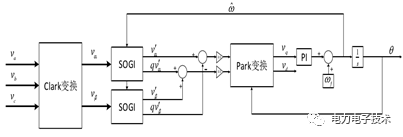系统结构二阶广义积分器是一种基于输入正弦信号 90°相角偏移的解决方案。输入电压信号通过 SOGI 后可以产生两相正交信号，一路输出信号跟踪输入电压信号，另一路信号实现对输入电压信号的 90°相角偏移。二、仿真模型及仿真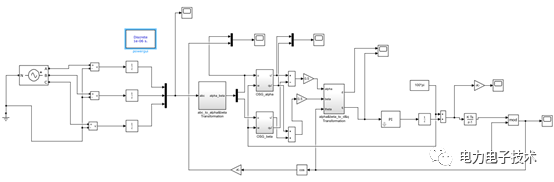系统总体仿真模型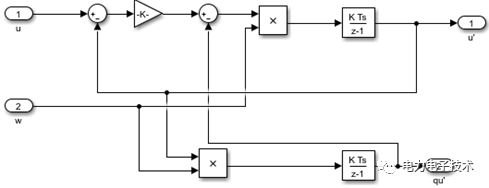OSG模块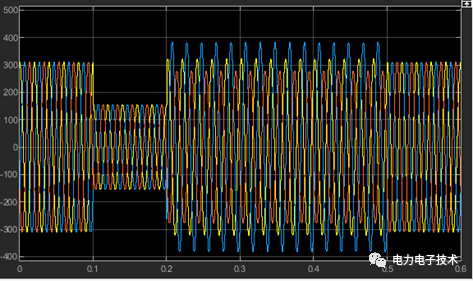三相电网模拟源波形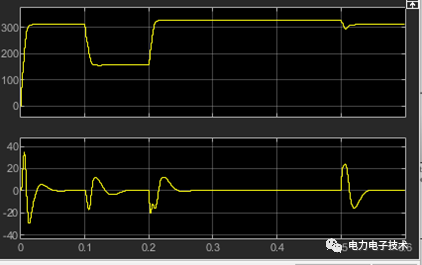正序电压Ud、Uq波形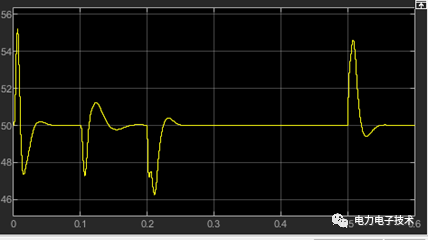锁相频率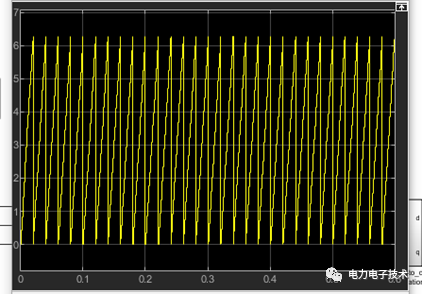锁相角度随着电力电子产品被大量应用于人类的生活之中，谐波污染问题也日趋严重。电力有源滤波器作为一种谐波污染治理解决方案也被广泛应用。作为其核心技术之一的锁相环技术也逐渐引起了众多专家和学者的关注，越来越多的锁相环技术不断被提出，针对各种环境下的锁相环也层出不穷。二阶广义积分器是一种基于输入正弦信号 90°相角偏移的解决方案。输入电压信号通过 SOGI 后可以产生两相正交信号，一路输出信号跟踪输入电压信号，另一路信号实现对输入电压信号的 90°相角偏移。通过上述仿真结果可以看出基于二阶广义积分软件锁相环仿真的正确性和有效性。

展开全文• 老师给的课程设计范例，看得不是很明白，但还是上传给大家看看
• 文章介绍了一种二阶数字锁相环的基本原理和其基于FPGA的实现方案,详细阐述了鉴相器、环路滤波器和数控振荡器等环路部件的参数设计及电路结构,并引入一种有效的环路状态检测方法,仿真验证了设计的正确性和有效性。
• 二阶锁相环环路滤波器的matla设计代码，自定义阻尼系数（默认为0.707）和噪声带宽，给出滤波器参数值。
• 详细介绍二阶RC有源滤波器的设计设计，对在锁相环中应用深有启发
写在前面的话：本篇文章参考自杜勇工程师的《锁相环技术原理及FPGA实现》
目录二阶环的FPGA实现（基于quartus+matlab）一.依据模拟环设计数字环1.1从模拟到数字——双线性变换1.2 环路滤波器的数字化1.3 理想二阶环的参数设计1.4 理想二阶环的$Verilog HDL$设计二.二阶环路滤波器仿真相关结论
二阶环的FPGA实现（基于quartus+matlab）
$\quad$$\quad$在前面的文章中分析了的心脏——环路滤波器，也分析了一阶环的FPGA实现，这篇文章讨论的是二阶环的FPGA实现。
一.依据模拟环设计数字环
$\quad$$\quad$根据信号与系统的分析理论，一个系统完全由系统函数来确定，因此我们可从系统函数的角度出发，找到模拟电路与数字电路的转换关系，最终根据环路滤波器的数字域系统函数进行数字化设计。
1.1从模拟到数字——双线性变换
$\quad$$\quad$连续时间系统H(s)的极点有两种情况：单重节点和多重节点。但是一个多重节点环节可以看成由多个单重极点环节级联构成。例如，对二重极点的系统，有$H(s)=\frac{A}{(s-p)^2}=\frac{\sqrt{A}}{s-p}\frac{\sqrt{A}}{s-p}$
因此，可以将一阶环节$\frac{\sqrt{A}}{s-p}=\frac{K_a}{s-p}$
看成构成$H(s)$的最基本环节，其中，$K_a$为基本环节的增益。它对应于一阶微分方程$\frac{dy(t)}{dt}-py(t)=K_ax(t)$
其系统结构如图1所示。对该系统离散化，主要是对系统中的积分运算离散化。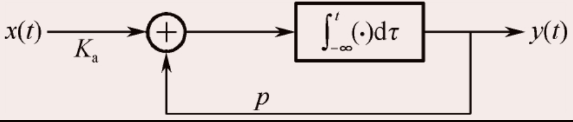$图1$
$\quad \quad$一次积分运算可以用梯形作数值计算，即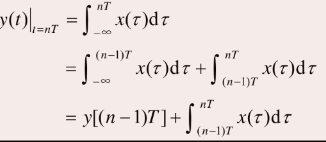将上式第二行的积分用梯形法近似，则有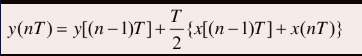该式为一次积分运算离散化后的数值计算公式，其中的T为采样间隔。将自变量中的符号$T$隐去，可写成差分方程的习惯表示形式$y(n)=y(n-1)+\frac{T}{2}[x(n-1)+x(n)]$
两边取单边$z$变换，并考虑到当$y(n)=0，n＜0$有
$Y(z)=z^{-1}+\frac{T}{2}[z^{-1}X(z)+X(z)]$
对上式进行整理，得到一阶环节的离散系统函数$H_1(z)=\frac {Y(z)}{X(z)}=\frac{T}{2}\frac{1+z^{-1}}{1-z^{-1}}$
也就是说，一次积分单元离散后，是上式描述的离散系统。
$\quad\quad$对连续一阶系统离散化后，可以得到其系统结构如下图所示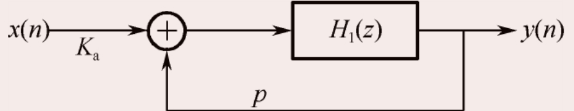$\quad\quad$根据上图，可求得离散的系统函数为$H_i(z)=\frac{K_a H_1(z)}{1-pH_1(z)}$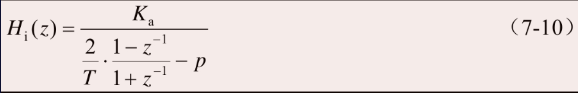$\quad\quad$将此式与连续的一阶环路做对比，得出连续时间系统和离散时间系统之间的转换公式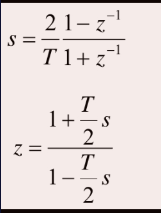1.2 环路滤波器的数字化
$\quad\quad$有了系统函数转换表达式，即可获得各种模拟环路滤波器所对应的数字化系统函数表达式，进而获取其数字化实现结构。
$\quad\quad$对于有源比例环路积分滤波器（理想积分滤波器）来讲，其数字化系统函数为
$F(z)=\frac{1+s\tau_2}{s\tau_1}=\frac{2\tau_2+T}{2\tau_1}+\frac{T}{\tau_1}\frac{z^{-1}}{1-z^{-1}}$
$\quad\quad$由于在二阶锁相环路中，理想二阶环路具有共他两种环路无法比拟的优异性能，因此接下来主要讨论这种环路滤波器及其构成的锁相环路。
$\quad\quad$对于上式，令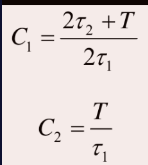则上式变换为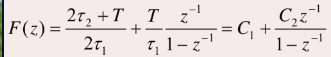其对应的系统结构可用下图来表示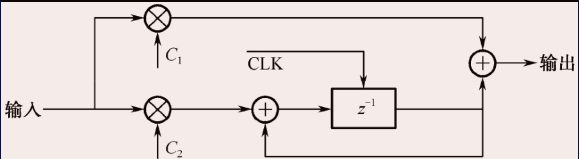1.3 理想二阶环的参数设计
各项参数如下：

软件：Quartus prime 18.0
FPGA系统工作时钟速率=系统采样频率$f_s$=$8 kHz$
数字震荡器固有频率$f_o=400Hz$
输入为10bit二进制补码数据
输出为10bit二进制补码数据
鉴相乘法器输出位宽：19比特
鉴相滤波器系数位宽：8比特
鉴相滤波器输出数据位宽：30比特
环路增益K=34 Hz
NCO频率字位宽：30比特
NCO相位字位宽：35比特

$\quad\quad$为便于比较，我们仍根据一阶环实例要求进行设计。根据前面的分析，理想二阶环的FPGA实现过程，不过是在一阶环的基础上增加一个环路滤波器功能模块而已。
$\quad\quad$根据前面的推导，求取环路滤波器系数C1、C2的值，需要获取采样周期$T$，以及滤波器时间常数$τ_1$、$τ_2$的值。由于系统采样频率$f_s=8000Hz$，$T=1/f_s=1/8000$s。需要注意的是，$τ_1$、$τ_2$是典型的模拟环路滤波器参数，这也是为什么我们将目前的方法称为依据模拟环设计数字环的原因。
$\quad\quad$如何计算$τ_1$、$τ_2$？根据第前面关于环路滤波器的讨论，从环路相位裕度参数出发设计这两个参数，而相位裕度与阻尼系数ξ直接相关。根据图6-38的仿真结论，一般取阻尼系数ξ=0.7。对于理想二阶环来讲$ω_n=\sqrt{\frac{K}{\tau_1}}$$ξ=\frac{\tau_2}{2}\sqrt{\frac{K}{\tau_1}}$
$\quad\quad$注意，在上式中，$K$的单位为$rad/s$，$ω_n$的单位为$rad/s$。现在，我们已经获取了环路增益参数（$K=34 Hz=213.6283 rad/s$）、阻尼系数（$ξ=0.7$），还需要获取一个参数，比如$τ_1、τ_2$之间的关系，才能计算出最终的时间常数值，进而计算出$C1、C2$的值。
$\quad\quad$根据模拟环路的性能分析，环路滤波器$3dB$截止带宽的大小直接影响到$VCO$输出的信号质量，要计算出$τ_1、τ_2$之间的关系，需要首先确定环路滤波器3dB截止带宽的$f_c$大小。根据前面的分析，取$f_c=10 Hz$（注意，公式中的截止频率单位为Hz），则有$10=\sqrt{\frac{2}{{2}{\tau_1^2-2\tau_2^2}}}$
$\quad\quad$结合前面的公式，带入$K,ξ$的值，得到$\tau_1,\tau_2$的值，再根据$τ_1、τ_2$的值，分别计算环路滤波器系数C1，C2。
1.4 理想二阶环的$Verilog HDL$设计
$\quad$这里只介绍二阶环的环路滤波器的$verliog$ 设计，由前面推导得到的二阶锁相环的Z域公式，将Z域公式转换到时域中，才能转换为我们可以用的形式。$F(z)=\frac{Y(z)}{X(z)}=C_1+\frac{C_2 z^{-1}}{1-z^{-1}}$
$\quad$令$\frac{C_2 z^{-1}}{1-z^{-1}}X(z)=D(z)$
由$z$变换的性质，上式的时域表达式为：$C_2x(n)+d(n)=d(n+1)$
结合整个$Z$域表达式,可得出其最终的时域表达式为：$y(n)=C_1x(n)+d(n)$具体实现代码如下：
/*

MODULE DECLARATION

*/
module LoopFilter(
rst,
clk,
pd,
frequency_df
);

/*

FUNCTION DECLARATION

*/

/*

LOCAL PARAMETER

*/

/*

PORT DECLARATION

*/
input  rst;                              //复位信号，高电平有效
input  clk;                              //FPGA系统时钟：8 kHz
input  signed [29:0]  pd;                // 输入数据：8 kHz，数据源来自fir滤波器的输出
output signed [29:0]  frequency_df;      // 环路滤波器输出数据

/*

REG & WIRE DECLARATION

*/
reg signed[29:0]sum_d;
wire signed[29:0]pd_c2,pd_c1,sum;

/ACHIEVEMENT

assign pd_c1={{1{pd}},pd[29:1]};//C1
assign pd_c2={{9{pd}},pd[29:9]};//C2

always @(posedge clk or posedge rst)
if (rst)
sum_d <= 0;
else
sum_d <= sum;

assign sum = pd_c2 + sum_d;
assign frequency_df = sum_d + pd_c1;

endmodule

这里需要说明的是，为了简化运算，C1和C2以移位代替了乘法，所以数值采用了近似的方法。
$\quad$再结合一阶环中的其他代码，就可以得到完整的二阶环$verliog$代码。
二.二阶环路滤波器仿真相关结论
$\quad$经过对二阶环的仿真，我们得出了下面的结论：

理想二阶环路增益直接影响环路的锁定性能，当环路参数设定后，环路的最大增益就确定了，当增益超过这个值时，环路不能锁定。
环路能够正常锁定的情况下，增益越大，锁定时间越大，锁定速度越快。
理想二阶环路的捕获带宽在工程设计中是有限的。
仅改变环路增益，对捕获带宽的影响不大。


展开全文• 在分析Bussgang类恒模算法(Constant Modulus Algorithm,CMA）盲均衡基础上，使用改进型CMA结合二阶锁相环（PLL）的盲均衡方法，实现对MQAM（M=4,16,64）和MPSK（M=2,4,8）信号经过高斯噪声信道后发生的频率偏移和...
• 锁相环设计、仿真与应用(第5版) 基本信息 * 作者： (美)Roland E.Best * 译者： 李永明[同译者作品] * 丛书名： 国外大学优秀教材--微电子类系列(翻译版) * 出版社：清华大学出版社 * ISBN：9787302128823 ...
• 锁相环设计、仿真与应用(第5版) 基本信息 * 作者： (美)Roland E.Best * 译者： 李永明[同译者作品] * 丛书名： 国外大学优秀教材--微电子类系列(翻译版) * 出版社：清华大学出版社 * ISBN：9787302128823 ...
• 锁相环作为集成电路中关键的模块，被广泛地应用于各领域中。...锁相环的闭环传递函数表达为：将式(2)整理为标准二阶系统传递函数：对此闭环系统的输入施加X(s)=1/s的阶跃信号时，得到输出信号：对式(6)...
• 导读：本文在论述了电荷泵锁相环基本原理的基础上，对有源环路滤波器的结构以及滤波器对锁相环性能的影响进行了分析，推导出有源环路滤波器参数的设计方法。 　电荷泵结构的锁相环（CPLL）具有易于集成、低功耗、无...
• 理想二阶设计公式 经过本章对锁相环路的讨论，尤其环路噪声性能的讨论，环路设计参数的公式已基本讨论完毕。在通信系统中，克服系统噪声是无法避免的问题，也是通信系统设计的难点和重点。因此，大多数锁相环路均...
• 针对GPS接收机载波跟踪环环宽与跟踪的动态性能问题，在分析影响GPS信号...并使系统性能达到最佳的问题，即使用环宽为18 Hz的二阶锁相环辅助环宽为10 Hz的三阶锁频环可以跟踪动态范围小于10 g、100 g/s的高动态信号。
• 以及仿真测试过程和具体技术细节，主要包括设计平台及开发环境介绍、锁相环跟踪相位的原理、FPGA实现数字信号处理基础、锁相环路模型、一阶环路的FPGA实现、环路滤波器与锁相环特性、二阶环路的FPGA实现、锁相环路...
• 载波跟踪环路设计是GPS 接收机中的关键技术，载波环鉴别器的类型确定了跟踪环的类型，为了有效地防止因为数据跳变引起的鉴别误差，并且使其频率鉴别范围大，精度高，采用一种二阶锁频环( FLL) 辅助三阶锁相环( PLL) ...